DAX Time Intelligence Explained - Excelerator BI

# DAX Time Intelligence Explained

Level: Beginners

I help a lot of people on forums who ask questions about time intelligence for DAX.  If you are just starting out then the chances are that you may not even be clear what time intelligence is and hence sometimes you don’t even know what to ask.  Often the question is something like “I want to show this year and last year on a chart”, or “total year to date this year compared with last year” etc. If you want to do any time based comparison using DAX, Power Pivot and or Power BI, then this article explaining time intelligence is the right article for you.

## Definition of Time Intelligence

Time intelligence is the collective name for a set of patterns (DAX in this case) that can be used to solve time comparison problems.  Examples include comparing:

• Same period prior month, quarter, year etc.
• Same period next month, quarter, year etc.
• Same period year to date compared with prior year, next year etc.
• Rolling 30 days, 60 days, 90 days, 12 months etc.
• etc. – there are many many more

Time intelligence is used when you want to “time shift” any period with another period of time for comparison purposes or to simply display a different period than the selection.## Understanding Filter Context

Before you can understand why time intelligence needs a special approach in DAX, you first need to have a clear understanding of Filter Context.

Pivot Tables and Power BI visuals both slice data so that you can “drill” and “filter” to see a sub-set of data.  Take the image shown below.  On the left is a pivot table and on the right is a Power BI visual.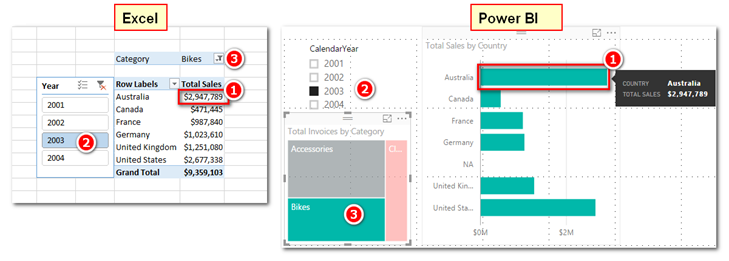Starting with the Excel Pivot table on the left, every value cell in the pivot table has been filtered more or less by the Rows, Columns, Filters and Slicers that make up the Pivot Table.  The cell highlighted as 1 (above left) has the following filters applied.

• Territory[Country] = “Australia” – this comes from the Rows in the Pivot
• ‘Calendar'[Year] = “2003” – this comes from the Year in the slicer
• Product[Category] = “Bikes” – this comes from the Category in the filter.

After these filters are applied, the calculation for the cell is evaluated and the answer \$2,947,789 is returned to the cell.  Every value cell in the pivot table is evaluated in exactly the same way – including the Grand Total row in the pivot table.  In the case of the grand total row, Product[Category] and ‘Calendar'[Year] have the same filters, but there is no filter on Territory[Country].

On the right hand side in the image above is a Power BI visual.  Filtering in Power BI visuals fundamentally works the same way as a pivot table however there are more places for cross filtering to come from.  In the image above, the same filtering is applied as in the Pivot table but in the Power BI example the filters are applied in a different way.

• Territory[Country] = “Australia” – this comes from the bar chart Axis
• ‘Calendar'[Year] = “2003” – this comes from the Year in the slicer
• Product[Category] = “Bikes” – this comes from the Category in the tree map visual.

When filter context is passed from a visual to the underlying data model, all the relevant tables are filtered before the calculation is completed.  Filter first, evaluate second is a fundamental principle for all DAX formulas.

## The Time Intelligence “Problem”

Let’s assume you want to compare total sales on a particular year vs prior year.  One way to do this (in Excel) is to put the years onto Columns in a pivot table as shown below (a similar approach can be used in Power BI).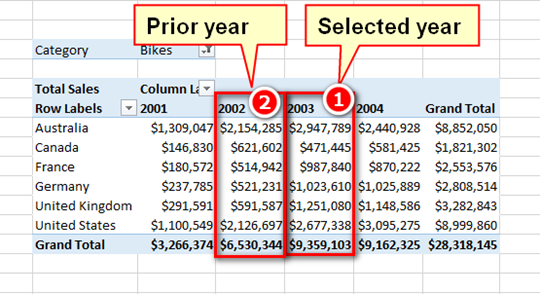But doing it this way causes many problems, including:

• There are years in the pivot table that you don’t want (eg 2001, 2004).  You will need to somehow manually hide or filter the ones you don’t need.
• If you want to calculate the absolute change or % change year on year you will need to hard code some formulas in the cells next to the spreadsheet and hence they can’t be reused in other visuals later.
• If you want to look at a different year you will potentially have to go through the process of doing the filtering and formulas again from scratch.

A better way to solve this problem is to select the current period (using a slicer or filter of some sort) and have a universal formula that returns the result relative to the selection.  So if you select 2003, the formula will automatically select 2002 for you.  If you select 2002, it will automatically select 2001 (and so on).

### Filtering is Now Your Enemy

If you want to write a relative time intelligence formula, then the natural filtering behaviour of a visual becomes your enemy. Imagine a calendar table with a filter applied Calendar[Year] = 2003.  If you imagine what the filtered data model would look like “Under the hood”, it should be clear that the filtered calendar table will show rows starting on 1 Jan 2003 and ending on 31 Dec 2003 – it will contain 365 unique days for the single year 2003.  The filter has already been applied to the data model so only days in 2003 are available for the calculation – how then can the data model possibly access sales for the year 2002?  There needs to be a way to go back in time and fetch a different period (in this case the period is 1 year earlier in time than the selected period), yet the 2003 filter has already been applied preventing this from occurring naturally.  This is the reason why there needs to be a special set of time intelligence functions – to solve this natural filtering “problem”.

### How Time Intelligence Functions Work

Time intelligence functions are specifically designed to solve the filtering issue described above.  All time intelligence functions execute the following 4 steps:

1. first “detect” the current filter context to determine what the “current” selected period is
2. then remove the calendar filtering from the underlying tables so that data for “all time” is once again available.
3. then perform a time shift to find a different period in time (as specified in the formula).
4. Finally reapply filters on the data model for the time shifted period prior to calculating the final value.## Custom vs. Inbuilt Time Intelligence

There are 2 types of time intelligence functions – Custom and Inbuilt.  Inbuilt time intelligence functions are easier to write because they have been designed to protect the user (ie you) from the complexity.  I am not going to cover Inbuilt time intelligence in depth here because they are relatively easy to use.  See link at the bottom of the page if you would like to download the DAX reference guide I produced (including all the time intelligence functions).

The reason inbuilt time intelligence functions are easier to learn is they actually are what is known as “Syntax Sugar”.  Microsoft has created these special functions to make them easier to write and easier to understand.  You follow the simple syntax and Power Pivot does the rest.  But under the hood the inbuilt time intelligence functions are converted to the full syntax prior to evaluation.  Consider the following two examples (just to illustrate the potential complexity in the custom version).

`Total Sales Year to Date (inbuilt) = TOTALYTD([Total Sales], 'Calendar'[Date])`
```Total Sales Year to Date (custom) =
CALCULATE(
[Total Sales],
FILTER(
ALL('Calendar'),
'Calendar'[Year] = MAX('Calendar'[Year])
&& 'Calendar'[Date] <= MAX('Calendar'[Date])
)
)
```

Both of these formulas return the exact same result, and in fact both use the same approach to calculating the result under the hood.  The only difference is the inbuilt version is much easy to write because you (the DAX author) are protected from the full syntax.

The end result (regardless which formula is used) looks like this in a Pivot Table.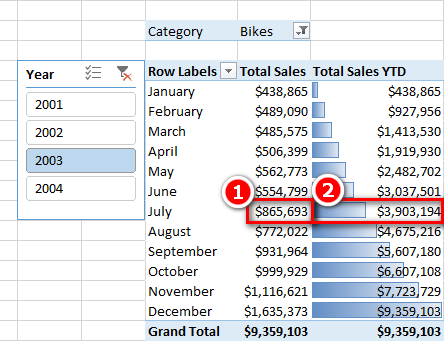Looking at the highlighted cells, even though cell 1 above is filtered to show only sales for the month of July 2003, the Time Intelligence function (cell 2 above) is able to display sales for the period Jan – Jul 2003.  It does this because the formula performs the following 4 steps.

1. It first “detects” the current filter context to determine what the “current” selected period is.  In this case July 2003.
2. It then removes the Calendar table filtering from the underlying tables so that all data is once again available.
3. It then performs a time shift to find a different period in time – in this case it holds the end date in the current filter context the same (31 July 2003) but shifts the start date back to 1 Jan 2003.
4. Finally it reapplies filters on the data model for the time shifted period prior to calculating the final value.

## How to Read a Custom Time Intelligence Formula

The custom time intelligence formulas can be daunting when you first see them – but actually they are easy to understand as long as you clearly understand the role of each part of the formula.  Below is the same formula again (from above) to make it easier for me to refer to.

```Total Sales Year to Date (custom) =
CALCULATE(
[Total Sales],
FILTER(
ALL('Calendar'),
'Calendar'[Year] = MAX('Calendar'[Year])
&& 'Calendar'[Date] <= MAX('Calendar'[Date])
)
)
```

Line 2 (CALCULATE) is the only function that can change the natural filtering behaviour coming from a visual – that’s what it does.   CALCULATE always filters first, evaluates second (as mentioned above).  So lines 4 – 8 are executed first (filters applied) and then the formula on line 3 is evaluated last.

Lines 4 – 8 (FILTER) is where the filtering occurs.  There are a few confusing things here.

• Line 5 refers to ALL(‘Calendar’) instead of simply ‘Calendar’.
• Lines 6 and 7 seem to be evaluating against themselves – very confusing.
• Line 7 starts with a double ampersand &&

Let me explain each line.

### Line 5 ALL(‘Calendar’)

The key thing to understand here is that the filter portion of calculate always operates in the current filter context.  That means that the Calendar table in line 5 has already been filtered by the visual (eg the Pivot Table).  Looking back at the pivot table image above, this means that the line 5 is already filtered by the pivot table and hence the Calendar only has dates from 1 July 2003 until 31 July 2003 available – all other dates have been filtered away.  The purpose of the ALL Function therefore is to remove all filters from the current filter context.  If it didn’t remove these filters, it would not be possible to access dates outside of the month of July 2003.

Now they have all been removed, it is time to add back the date filters that are needed.

### Line 6 MAX( )

Line 6 reads “where ‘Calendar'[Year] = MAX(‘Calendar'[Year])” and hence it seems to be referring to itself. The way to read and understand line 6 (and 7) is as follows:

• Whenever you see an aggregation function (in this case MAX) around a column, it means “go and read the value from the current filter context”.
• Whenever you see a “naked” reference to a column (ie there is no aggregation function around the column), then it means “go and apply a new filter on this column in the data model.

Taking these 2 rules, it should be clear that MAX(‘Calendar'[Year]) in the current filter context is = 2003.  Line 6 therefore says “Go and apply a new filter on ‘Calendar'[Year] to be equal to 2003.

Note that you can use any aggregation function in these formulas that does the job.  In lines 6 and 7, you could use SUM, MIN, MAX, AVERAGE or any other similar aggregation function and still get the same result.  You could also use VALUES or DISTINCT in the case of line 6, and LASTDATE in the case of line 7.  There is no right or wrong answer, simply think about the need and then find the best function to use.

### Line 7 && and MAX( )

Line 7 reads “and ‘Calendar'[Date] <= MAX(‘Calendar'[Date])”.  The double ampersand && is the inline syntax for a logical AND.  Using this knowledge and the same logic as with line 6, the way to read line 7 is as follows:

“AND also go and apply another new filter this time on ‘Calendar'[Date] to be less than or equal to 31 July 2003”.

With both of these filters applied in a logical AND, the Calendar table will contain all of the dates from 1 Jan 2003 until 31 July 2003.

## The Result

Once the time intelligence function has been written, it can be added to a visual as shown below (Power BI Desktop).  Note how the YTD formula seems to “defy” the filter context coming from the visualisation due to the custom time intelligence function that has been written and explained.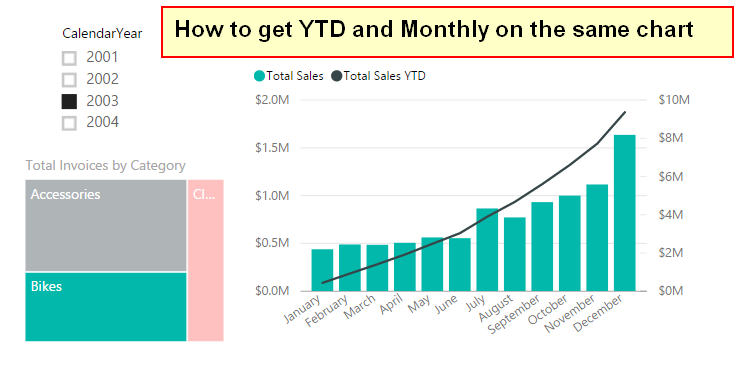## The Trouble with Syntax Sugar

Syntax sugar is great as it makes otherwise hard formulas very easy to write with a limited understanding of filter context, row context, filter propagation, context transition etc.  There are a few down sides however.  Firstly is that you don’t get to learn these very important concepts and hence you are delayed in becoming a true Power Pivot and Power BI Ninja.  Secondly the inbuilt time intelligence functions only work in certain circumstances where you have a day level Gregorian calendar.  Read more about that here https://exceleratorbi.com.au/power-pivot-calendar-tables/

## Granularity

I personally hardly ever use the inbuilt time intelligence functions unless my data is at a day level of granularity (which is rare), and I find the custom functions become easy to write with practice.  Custom time intelligence functions become much more important when your data is not at a day level of granularity.  Most of the work I do for clients is either weekly or monthly data.  If you are in this situation you could “trick” Power Pivot that you are using daily data by loading all data in any given week or month on the same date (eg last day of the month) and use inbuilt time intelligence however this is hardly best practice.  A much better approach I think is to write custom time intelligence functions as outlined in this article.  If you are going down the  path of writing custom time intelligence, you should read my advice about adding an ID column into a Calendar table to make custom time intelligence functions easier to write.  https://exceleratorbi.com.au/power-pivot-calendar-tables/

## Sales vs Prior Year

Time for a different example.  Now that I have covered how a custom time intelligence function works, let me show you a couple of inbuilt time intelligence measures that solve the original problem (Sales vs Prior Year).

`Sales Prior Year 1 = CALCULATE([Total Sales], SAMEPERIODLASTYEAR('Calendar'[Date]))`
`Sales Prior Year 2 = CALCULATE([Total Sales], DATESADD('Calendar'[Date],-1,YEAR))`

Both of the above formulas use inbuilt time intelligence functions (shown in bold), but they also use CALCULATE.  Now you have an understanding that CALCULATE is performing a time shift, it should be much easier to understand what is happening in these formulas.  Both of these formulas in bold produce a table of dates that has been time shifted back by 1 year.  CALCULATE then takes this new table of dates, removes the current filter context from the Calendar table and then moves back in time by 1 year before reapplying the filter context and then doing the calculation.  One you have [Sales Prior Year] it is easy to write:

`Change vs Prior Year = [Total Sales] - [Sales Prior Year]`
`%Change vs Prior Year = DIVIDE([Change vs Prior Year], [Sales Prior Year])`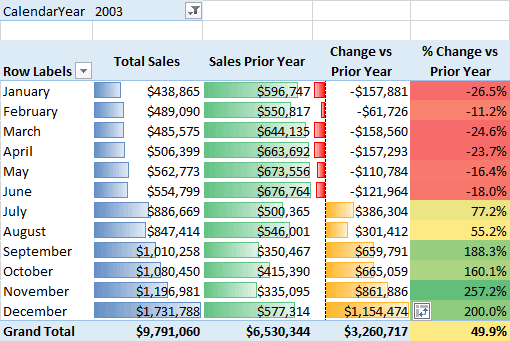So where can you find a list of all the inbuilt time intelligence functions?

## A Free DAX Reference Guide

One of my students at a recent training class asked me if there was a list of all DAX Functions – kind of like a cheat sheet.  I wasn’t able to find such a thing so I produced exactly that and I am making it available free to anyone that would like a copy here.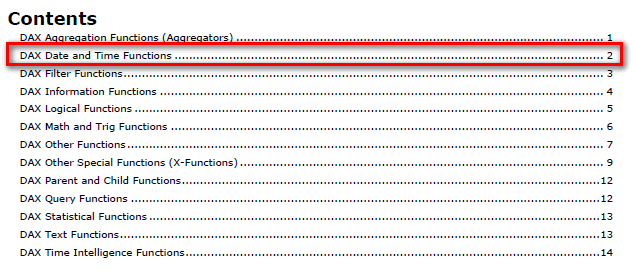This reference guide covers all of the inbuilt time intelligence functions on page 14 as well as every other function across the language all nicely laid out to make them easy to find.  You can download this reference guide below.  If you haven’t ready done so, why not sign up for my weekly newsletters at the same time so you are kept up to date with my latest tips and tricks about Power Pivot, Power Query and Power BI.